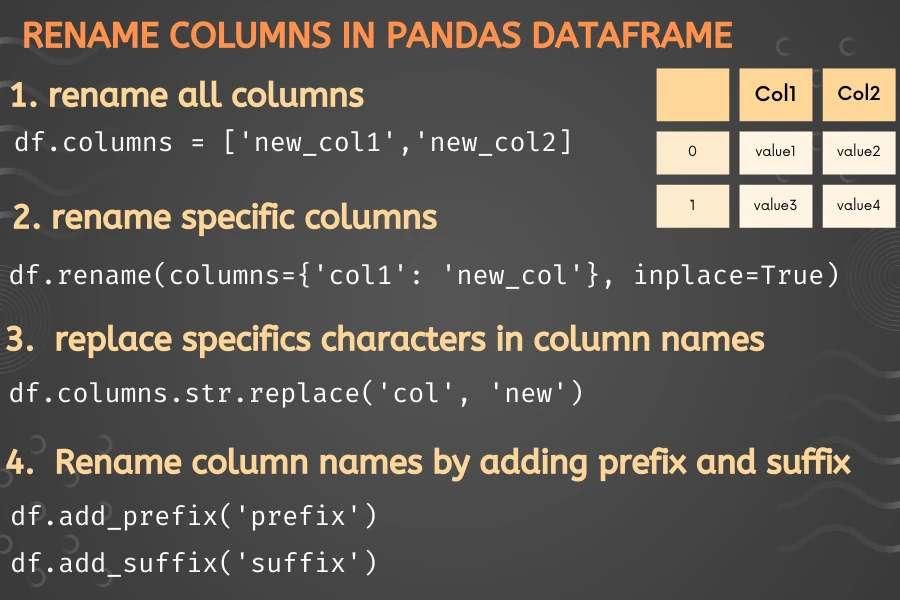# Simple ways to rename column names in pandas DataFrame

pandas DataFrame provides various functions such as `rename()`, `set_axis()`, `add_prefix()`, and `add_suffix()` to rename the columns of the DataFrame. You can rename either all columns or specific columns using these functions.### Rename all columns

You can rename all columns of a DataFrame using the pandas DataFrame `columns` attribute. This method is also used for setting column names if DatFrame does not have column names.

``````# create a DataFrame
import pandas as pd
df = pd.DataFrame({'name':['A', 'B'], 'age':[25, 30]})
# view DataFrame
df
# output
name  age
0    A   25
1    B   30

# rename all columns
df.columns = ['first_name', 'age_years']
# view updated DataFrame
df
# output
first_name  age_years
0          A         25
1          B         30

``````

Similarly, you can also use the `set_axis()` function of pandas DataFrame for renaming the column. Set the axis parameter to `columns` or `1` to rename the columns.

``````df.set_axis(['first_name', 'age_years'], axis='columns', inplace=True)
``````

### Rename specific column names

Sometimes, we want to rename specific columns of a pandas DataFrame. To rename specific columns of DataFrame, you can use the pandas DataFrame `rename()` function. The rename function takes a dictionary with old column names as keys and new column names as values.

``````# create a DataFrame
import pandas as pd
df = pd.DataFrame({'name':['A', 'B'], 'age':[25, 30], 'height': [5, 5.5]})

# rename name and height column
# use inplace=True to modify and update the current DataFrame
df.rename(columns={'name': 'first_name', 'height': 'height_feet'}, inplace=True)
# view updated DataFrame
df
# output
first_name  age  height_feet
0          A   25          5.0
1          B   30          5.5
``````

Similarly, you can also `dfply` package to rename the specific columns. `dfply` Python package is similar to R’s `dplyr` and supports the data manipulation with pipes on pandas DataFrame.

``````from dfply import *
# rename columns
# argument should be new column and parameter is old column name
df >> rename(first_name='name', height_feet='height')
``````

### Rename column names using `add_prefix()` and `add_suffix()` functions

Sometimes, you need to rename columns by adding some additional characters at the start or end of column names. In this case, you can use pandas DataFrame `add_prefix()` and `add_suffix()` functions.

``````# create a DataFrame
import pandas as pd
df = pd.DataFrame({'name':['A', 'B'], 'age':[25, 30], 'height': [5, 5.5]})

df
# output
f_name  f_age  f_height
0      A     25       5.0
1      B     30       5.5

# output
col1_S  col2_S  col3_S
0      A       1     0.1
1      B       2     0.2
``````

### Replace specific texts of column names

Sometimes, you would like to replace some specific characters in the column names. For example, if column names contain some special characters.

``````# create a DataFrame
import pandas as pd
df = pd.DataFrame({'name_':['A', 'B'], 'age_':[25, 30], 'height_': [5, 5.5]})
df
# output
name_  age_  height_
0     A    25      5.0
1     B    30      5.5

# replace _ with empty character
df.columns = df.columns.str.replace('_', '')
df
# output
name  age  height
0    A   25     5.0
1    B   30     5.5
``````